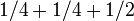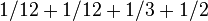There are finitely many finite groups with bounded number of conjugacy classes

Statement

In finite terms

Let$d$ be any positive integer. Then, the following hold:

1. There are only finitely many finite groups with exactly$d$ conjugacy classes of elements.
2. There are only finitely many finite groups with at most$d$ conjugacy classes of elements.
3. There exists a number$n_d$ dependent on$d$ such that any finite group of order more than$n_d$ has more than$d$ conjugacy classes.

In limit terms

Denote, for a finite group$G$, the number of conjugacy classes of$G$ by$n(G)$. Then:$\lim_{|G| \to \infty} n(G) = \infty$

Facts used

1. Size of conjugacy class equals index of centralizer (we use it to derive a primitive version of the class equation of a group)
2. Number of Egyptian fraction representations of unity with bounded number of fractions is finite: For fixed$d$, there are only finitely many solutions to:$\sum_{i=1}^d \frac{1}{a_i} = 1$

where the$a_i$ are (not necessarily distinct) positive integers.

Proof

We prove that there is a bound on the order of any finite group$G$ with exactly$d$ conjugacy classes.

For a finite group$G$ of order$n$ with$d$ conjugacy classes, let$a_1 \ge a_2 \ge \dots \ge a_d$ be the sizes of the centralizers of representatives of each of the conjugacy classes, arranged in descending order. By fact (1), the size of the$i^{th}$ conjuacy class is$|G|/a_i$. Since the group is a union of its conjugacy classes, we get:$\frac{|G|}{a_1} + \frac{|G|}{a_2} + \dots + \frac{|G|}{a_d} = |G|$

Canceling$|G|$ from both sides, we get:$\frac{1}{a_1} + \frac{1}{a_2} + \dots + \frac{1}{a_d} = 1$

Note that$a_1 = |G|$ so we can recover$|G|$ from the values of the$a_i$s. By fact (2), the number of positive integer solutions$(a_1,\dots,a_d)$ to the above system is finite. Taking the maximum of the possible values of$a_1$ among all these gives a finite upper bound on the order of$G$, and hence a finite limit on the number of possible$G$s that have$d$ conjugacy classes.

Particular cases

Note that there are a number of additional necessary (but not sufficient) conditions for a decomposition:$\sum_{i=1}^d \frac{1}{a_i} = 1$

to arise from a group, with$a_1 \ge a_2 \ge \dots a_d$. These include:

1. All the$a_i$s must divide$a_1$, since by Lagrange's theorem, any centralizer is a subgroup and hence must divide the order of the group.
2. The number of$a_i$s that equal$a_1$ also divides$a_1$ (because this number is the order of the center).
3. If the number of$a_i$s that equal$a_1$ is$\alpha$, then all the$a_i$s are multiples of$\alpha$. For a nontrivial group, all of them are strictly bigger than$\alpha$.

Below are some examples. The list of Egyptian fraction representations is exhaustive for$d=1,2,3$, but there are many other cases for$d = 4$ that we have not listed here.

Number of conjugacy classes$d$ Egyptian fraction representation of unity Groups where this representation is realized
1 1 trivial group
2$1/2 + 1/2$ cyclic group:Z2
3$1/3 + 1/3 + 1/3$ cyclic group:Z3
3$1/4 + 1/4 + 1/2$ not realized for any group -- condition (3) violated.
3$1/6 + 1/3 + 1/2$ symmetric group:S3
4$1/4 + 1/4 + 1/4 + 1/4$ cyclic group:Z4, Klein four-group
4$1/6 + 1/3 + 1/4 + 1/4$ not realized for any group -- condition (1) violated
4$1/6 + 1/6 + 1/3 + 1/3$ not realized for any group -- condition (3) violated
4$1/6 + 1/6 + 1/6 + 1/2$ not realized for any group -- condition (3) violated
4$1/8 + 1/8 + 1/4 + 1/2$ not realized for any group -- condition (3) violated
4$1/10 + 1/5 + 1/5 + 1/2$ dihedral group:D10
4$1/12 + 1/4 + 1/3 + 1/3$ alternating group:A4
4$1/12 + 1/12 + 1/3 + 1/2$ not realized for any group -- condition (3) violated